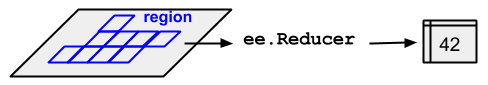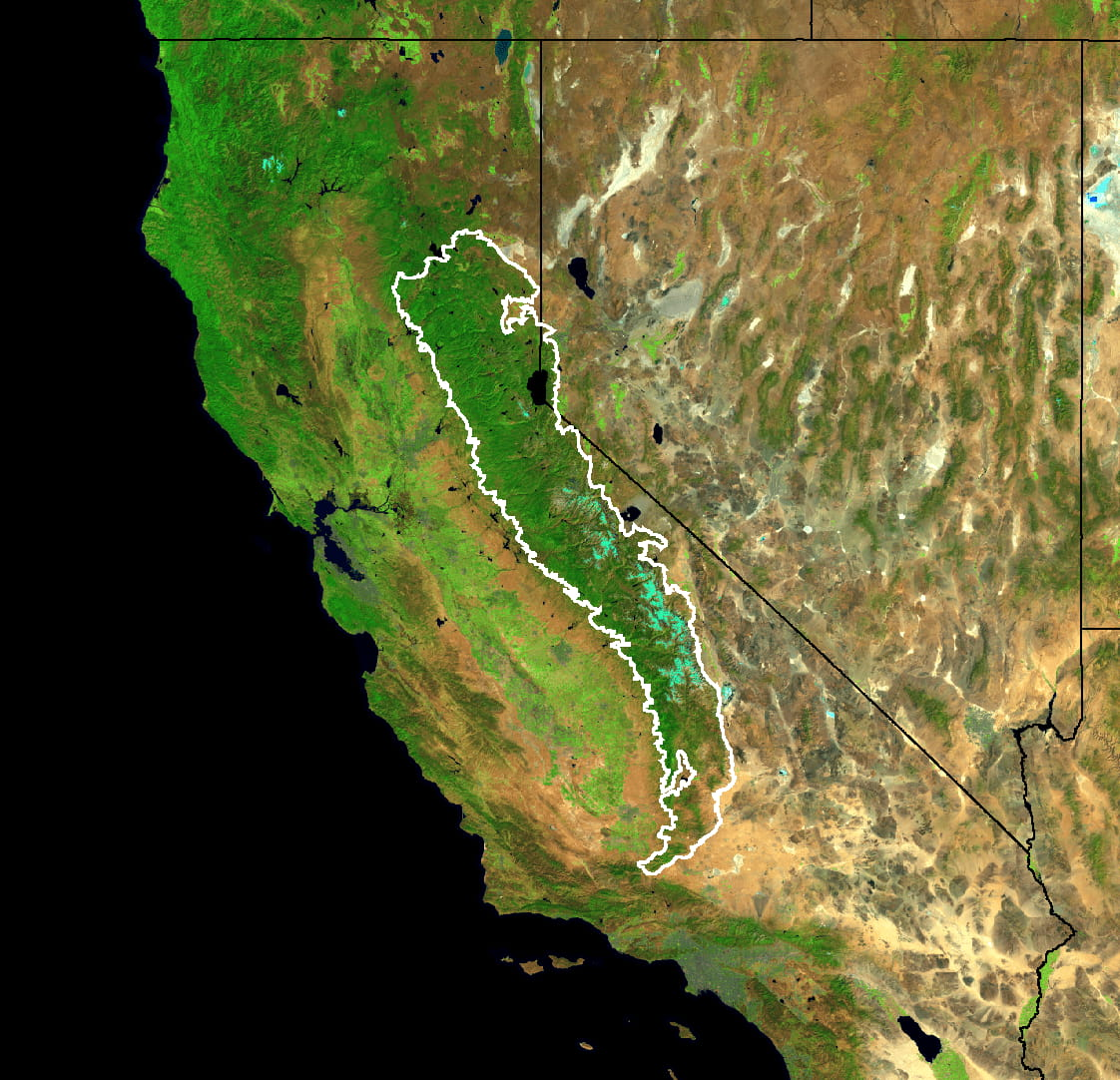Statistics of an Image Region

reduceRegion

To get statistics of pixel values in a region of an ee.Image, use image.reduceRegion(). This reduces all the pixels in the region(s) to a statistic or other compact representation of the pixel data in the region (e.g. histogram). The region is represented as a Geometry, which might be a polygon, containing many pixels, or it might be a single point, in which case there will only be one pixel in the region. In either case, as illustrated in Figure 1, the output is a statistic derived from the pixels in the region.Figure 1. An illustration of an ee.Reducer applied to an image and a region.

For an example of getting pixel statistics in a region of an image using reduceRegion(), consider finding the mean spectral values of a 5-year Landsat composite within the boundaries of the Sierra Nevada Coniferous Forest (illustrated by Figure 2):

// Load input imagery: Landsat 7 5-year composite.
var image = ee.Image('LANDSAT/LE7_TOA_5YEAR/2008_2012');

// Load an input region: Sierra Nevada.
var region = ee.Feature(ee.FeatureCollection('EPA/Ecoregions/2013/L3')
.first());

// Reduce the region. The region parameter is the Feature geometry.
var meanDictionary = image.reduceRegion({
reducer: ee.Reducer.mean(),
geometry: region.geometry(),
scale: 30,
maxPixels: 1e9
});

// The result is a Dictionary.  Print it.
print(meanDictionary);

To force the computation, it suffices to print the result, which the Code Editor will display as a Dictionary in the console. The output should look something like:

B1: 25.406029716816853
B2: 23.971497014238988
B3: 22.91059593763103
B4: 54.83164133293403
B5: 38.07655472573677
B6_VCID_2: 198.93216428012906
B7: 24.063261634961563Figure 2. False color composite of the Landsat image data for California and Nevada. The region over which to reduce is shown in white.

Note that in this example the reduction is specified by providing the reducer (ee.Reducer.mean()), the geometry (region.geometry()), the scale (30 meters) and maxPixels for the maximum number of pixels to input to the reducer. A scale should always be specified in reduceRegion() calls. This is because in complex processing flows, which may involve data from different sources with different scales, the scale of the output will not be unambiguously determined from the inputs. In that case, the scale defaults to 1 degree, which generally produces unsatisfactory results. See this page for more information about how Earth Engine handles scale.

There are two ways to set the scale: by specifying the scale parameter, or by specifying a CRS and CRS transform. (See the glossary for more information about CRS's and CRS transforms). For example, the meanDictionary reduction (above) is equivalent to the following:

// As an alternative to specifying scale, specify a CRS and a CRS transform.
// Make this array by constructing a 4326 projection at 30 meters,
// then copying the bounds of the composite, from composite.projection().
var affine = [0.00026949458523585647, 0, -180, 0, -0.00026949458523585647, 86.0000269494563];

// Perform the reduction, print the result.
print(image.reduceRegion({
reducer: ee.Reducer.mean(),
geometry: region.geometry(),
crs: 'EPSG:4326',
crsTransform: affine,
maxPixels: 1e9
}));

In general, specifying the scale is sufficient and results in more readable code. Earth Engine determines which pixels to input to the reducer by first rasterizing the region. If a scale is specified without a CRS, the region is rasterized in the image's native projection scaled to the specified resolution. If both a CRS and scale are specified, the region is rasterized based on them.

Pixels in the region

Pixels are determined to be in the region (and weighted) according to the following rules, applied in the specified scale and projection:

• Unweighted reducers (e.g. ee.Reducer.count() or ee.Reducer.mean().unweighted()): pixels are included if their centroid is in the region and the image's mask is non-zero.
• Weighted reducers (e.g. ee.Reducer.mean()): pixels are included if at least (approximately) 0.5% of the pixel is in the region and the image's mask is non-zero; their weight is the minimum of the image's mask and the (approximate) fraction of the pixel covered by the region.

The maxPixels parameter is needed to get the computation to succeed. If this parameter is left out of the example, an error is returned, which looks something like:

There are multiple options to get past these errors: increase maxPixels, as in the example, increase the scale, or set bestEffort to true, which automatically computes a new (larger) scale such that maxPixels is not exceeded. If you do not specify maxPixels, the default value is used.